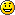# Finite fields

This topic introduces the algebraic concept of field. We shall give the precise definition of this algebraic structure and study the properties of finite fields. We shall find that in addition to the (infinite) field of real numbers that every schoolchild is familiar with, there exist many more. In cryptography, e. g. in encryption and computation of error-correcting codes, some of these fields have turned out to be very useful. On our way we shall also meet the algebraic structures ring and group.

Suggestion: before moving on with the following lessons, see “Divisibility and modular arithmetics”.$+$$\times$